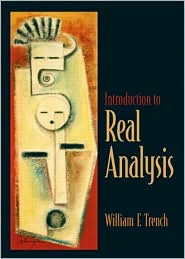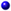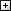Processing ......FreeComputerBooks.com Free Computer, Mathematics, Technical Books and Lecture Notes, etc.

Introduction to Real Analysis by William F. Trench
GIS Visualizer - You provide data, we visualize them on 40+ maps, 100% free!
• Title Introduction to Real Analysis
• Author(s) William F. Trench
• Publisher: Prentice Hall (December 14, 2002), William F. Trench (October 2009)
• Paperback: 574 pages
• Language: English
• ISBN-10: 0130457868
• ISBN-13: 978-0130457868Book Description

Using an extremely clear and informal approach, this book introduces readers to a rigorous understanding of mathematical analysis and presents challenging math concepts as clearly as possible. The real number system. Differential calculus of functions of one variable. Riemann integral functions of one variable. Integral calculus of real-valued functions. Metric Spaces. For those who want to gain an understanding of mathematical analysis and challenging mathematical concepts.

This is a text for a two-term course in introductory real analysis for junior or senior mathematics majors and engineering and science students with a serious interest in mathematics. Prospective educators of mathematically gifted high school students can also benefit from the mathematical maturity that can be gained from an introductory real analysis course.

The book is designed to fill the gaps left in the development of calculus as it is usually presented in an elementary course, and to provide the background required for insight into more advanced courses in pure and applied mathematics.

• Chapter 1 is concerned with the real number system. Section 1.1 begins with a brief discussion of the axioms for a complete ordered field, but no attempt is made to develop the reals from them; rather, it is assumed that the student is familiar with the consequences of these axioms, except for one: the completeness axiom. Since the difference between a rigorous and a nonrigorous treatment of calculus can be described largely in terms of the attitude taken toward completeness, I have expended considerable effort in developing its consequences. Section 1.2 is about mathematical induction. Although this may seem out of place in a real analysis course, I have found that the typical beginning real analysis student simply cannot do an induction proof without reviewing the method. Section 1.3 is devoted to elementary set theory and the topology of the real line, ending with the Heine-Borel and Bolzano-Weierstrass theorems.
• Chapter 2 covers the differential calculus of functions of one variable: limits, continuity, differentiability, 1'Hospital's rule, and Taylor's theorem. The emphasis is on rigorous presentation of principles; no attempt is made to develop the properties of specific elementary functions. Even though this may not be done rigorously in most contemporary calculus courses, I believe that the student's time is better spent on principles, rather than on reestablishing familiar formulas and relationships.
• Chapter 3 is devoted to the Riemann integral of functions of one variable. The integral is defined in the standard way in terms of Riemann sums. Upper and lower integrals are also defined there and used in Section 3.2 to study the existence of the integral. Section 3.3 is devoted to properties of the integral. Improper integrals are studied in Section 3.4. I believe that my treatment of improper integrals is more detailed than in most comparable .textbooks. A more advanced look at the existence of the proper Riemann integral is given in Section 3.5, which concludes with Lebesgue's existence criterion. This section can be omitted without compromising the student's preparedness for subsequent sections.
• Chapter 4 treats sequences and series. Sequences of constants are discussed in Section 4.1. I have chosen to make the concepts of limit inferior and limit superior parts of this development, mainly because this permits greater flexibility and generality, with little extra effort, in the study of infinite series. Section 4.2 provides a brief introduction to the way in which continuity and differentiability can be studied by means of sequences. Sections 4.3-4.5 treat infinite series of constants, sequences and infinite series of functions, and power series, again in more detail than in most comparable textbooks. The instructor who chooses not to cover these sections completely can omit the less standard topics without loss in subsequent sections.
• Chapter 5 is devoted to real-valued function of several variables. It begins with a discussion of the topology of Rn in Section 5.1. Continuity and differentiability are discussed in Sections 5.2 and 5.3. The chain rule and Taylor's theorem are discussed in Section 5.4.
• Chapter 6 covers the differential calculus of vector valued-functions of several variables. Section 6.1 reviews matrices, determinants, and linear transformations, which are integral parts of the differential calculus as presented here. In Section 6.2 the differential of a vector-valued function is defined as a certain linear transformation, and the chain rule is discussed in terms of composition of such functions. The inverse function theorem is the subject of Section 6.3, where the notion of branches of an inverse is introduced. In Section 6.4 the implicit function theorem is motivated by first considering linear transformations and then stated and proved in general.
• Chapter 7 covers the integral calculus of real-valued functions of several variables. Multiple integrals are defined in Section 7.1, first over rectangular parallelepipeds and then over more general sets. The discussion deals with the multiple integral of a function whose discontinuities form a set of Jordan content zero, over a set whose boundary has Jordan content zero. Section 7.2 deals with evaluation of multiple integrals by means of iterated integrals. Section 7.3 begins with the definition of Jordan measurability, followed by a derivation of the rule for change of content under a linear transformation, an intuitive formulation of the rule for change of variables in multiple integrals, and finally a careful statement and proof of the rule. The proof is complicated, but this is unavoidable.
• Chapter 8 deals with metric spaces. The concept and basic properties of a metric space are introduced in Section 8.1. Section 8.1 discusses compactness in a metric space, and Section 8.3 covers continuous functions on metric spaces.All CategoriesRecent BooksIT Research LibraryMiscellaneous BooksComputer LanguagesComputer ScienceData Science/DatabasesElectronic EngineeringJava and Java EE (J2EE)Linux and UnixMathematicsMicrosoft and .NETMobile ComputingNetworking and CommunicationsSoftware EngineeringSpecial TopicsWeb Programming